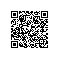# 驳“反驳老赵之“伪”递归”

public static readonly Func<int, int> fac = x => x <= 1 ? 1 : x * fac(x - 1);
static void Main(string[] args)
{
int x = 5;
Console.WriteLine(fac(x));
}

internal class Program
{
// Fields
public static readonly Func<int, int> fac;
// Methods
static Program()
{
if (CS$<>9__CachedAnonymousMethodDelegate1 == null) { CS$<>9__CachedAnonymousMethodDelegate1 = new Func<int, int>(null, (IntPtr) <.cctor>b__0);
}
fac = CS\$<>9__CachedAnonymousMethodDelegate1;
}
private static void Main(string[] args)
{
Console.WriteLine(fac.Invoke(5));
}
}

static int  Fac(int i)
{
return i <= 1 ? 1 : i * Fac(i - 1);
}

private static int Fac(int i)
{
return ((i <= 1) ? 1 : (i * Fac(i)));
}

# 总结使用钉钉扫一扫加入圈子
+ 订阅